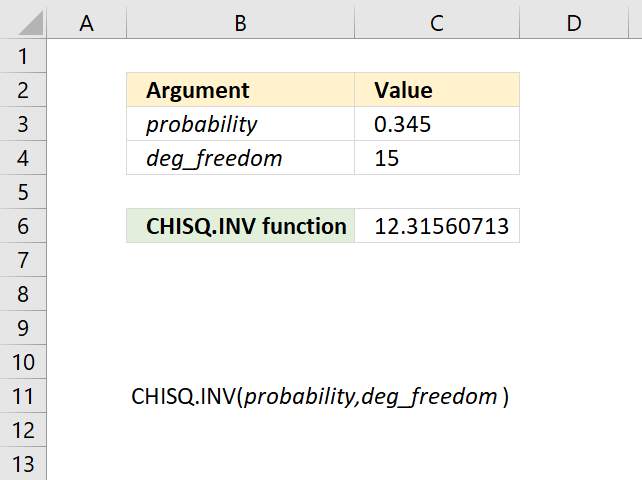Author: Oscar Cronquist Article last updated on September 28, 2018The CHISQ.INV function was introduced in Excel 2010 and calculates the inverse of the left-tailed probability of the chi-squared distribution. It has replaced the CHIINV function.

Formula in cell C6:

=CHISQ.INV(C3,C4)

### Excel Function Syntax

CHISQ.INV(probability,deg_freedom)

### Arguments

 probability Required. A numerical value representing the probability of the chi-squared function distribution. deg_freedom Required. A numerical value representing the degrees of freedom.